# SAT Math Multiple Choice Question 206: Answer and Explanation

### Test Information

Question: 206

11.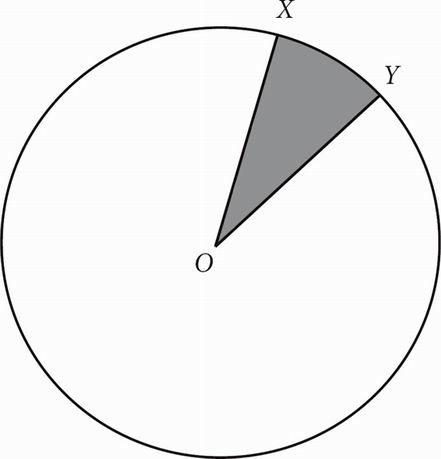In the figure above, O is the center of the circle and the diameter is 10. If the area of the shaded region is π, what is the length of minor arc XY ?

• A.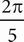• B.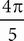• C.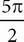• D. 5π

ASince the diameter is 10, the radius must be 5. A = πr2, so A = 25π. The shaded region takes up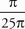or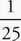of the area, so minor arc XY must take upof the circumference. C = πd so C = 10π. Therefore, the length of minor arc XY is(10π), or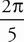, which is (A).